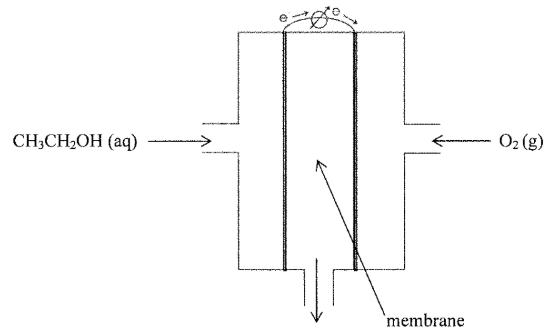# Problem: An illustration of an ethanol fuel cell is given below:The half reactions (written as reductions) for the fuel cell at 25 °C are given below.O2 (g) + 4 H3O+ (aq) + 4 e- → 6 H2O (l)                                                        ε°red = 1.23 VCH3COOH (aq) + 4 H3O+ (aq) + 4 e- → CH3CH2OH (aq) + 5 H2O (l)         ε°red = ???   acetic acid                                                 ethanolWrite the overall chemical reaction for the fuel cell, and list the product(s) released from the cell.

###### FREE Expert Solution

We’re being asked to write the overall chemical reaction for the fuel cell, and list the product(s) released from the cell.

Recall the mnemonics LEO GER.

Lose               Gain

Electron         Electrons

Oxidation       Reduction###### Problem Details

An illustration of an ethanol fuel cell is given below:The half reactions (written as reductions) for the fuel cell at 25 °C are given below.

O2 (g) + 4 H3O+ (aq) + 4 e- → 6 H2O (l)                                                        ε°red = 1.23 V

CH3COOH (aq) + 4 H3O+ (aq) + 4 e- → CH3CH2OH (aq) + 5 H2O (l)         ε°red = ???

acetic acid                                                 ethanol

Write the overall chemical reaction for the fuel cell, and list the product(s) released from the cell.